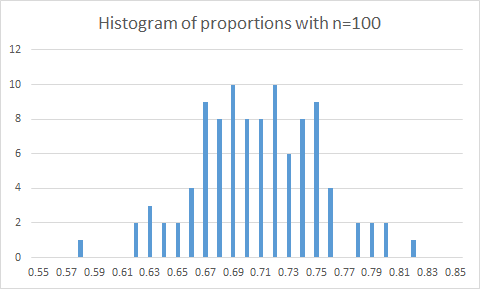24
Aug 16

## The pearls of AP Statistics 22

The law of large numbers - a bird's view

They say: In 1689, the Swiss mathematician Jacob Bernoulli proved that as the number of trials increases, the proportion of occurrences of any given outcome approaches a particular number (such as 1/6) in the long run. (Agresti and Franklin, p.213).

I say: The expression “law of large numbers” appears in the book 13 times, yet its meaning is never clearly explained. The closest approximation to the truth is the above sentence about Jacob Bernoulli. To see if this explanation works, tell it to your students and ask what they understood. To me, this is a clear case when withholding theory harms understanding.

Intuition comes first. I ask my students: if you flip a fair coin 100 times, what do you expect the proportion of ones to be? Absolutely everybody replies correctly, just the form of the answer may be different (50-50 or 0.5 or 50 out of 100). Then I ask: probably it will not be exactly 0.5 but if you flip the coin 1000 times, do you expect the proportion to be closer to 0.5? Everybody says: Yes. Next I ask: Suppose the coin is unfair and the probability of 1 appearing is 0.7. What would you expect the proportion to be close to in large samples? Most students come up with the right answer: 0.7. Congratulations, you have discovered what is called a law of large numbers!

Then we give a theoretical format to our little discovery.$p=0.7$ is a population parameter. Flipping a coin$n$ times we obtain observations$X_1,...,X_n$. The proportion of ones is the sample mean$\bar{X}=\frac{X_1+...+X_n}{n}$. The law of large numbers says two things: 1) as the sample size increases, the sample mean approaches the population mean. 2) At the same time, its variation about the population mean becomes smaller and smaller.

Part 1) is clear to everybody. To corroborate statement 2), I give two facts. Firstly, we know that the standard deviation of the sample mean is$\frac{\sigma}{\sqrt{n}}$. From this we see that as$n$ increases, the standard deviation of the sample mean decreases and the values of the sample mean become more and more concentrated around the population mean. We express this by saying that the sample mean converges to a spike. Secondly, I produce two histograms. With the sample size$n=100$, there are two modes (just 1o%) of the histogram at 0.69 and 0.72, while 0.7 was used as the population mean in my simulations. Besides, the spread of the values is large. With$n=1000$, the mode (27%) is at the true value 0.7, and the spread is low.Finally, we relate our little exercise to practical needs. In practice, the true mean is never known. But we can obtain a sample and calculate its mean. With a large sample size, the sample mean will be close to the truth. More generally, take any other population parameter, such as its standard deviation, and calculate the sample statistic that estimates it, such as the sample standard deviation. Again, the law of large numbers applies and the sample statistic will be close to the population parameter. The histograms have been obtained as explained here and here. Download the Excel file.